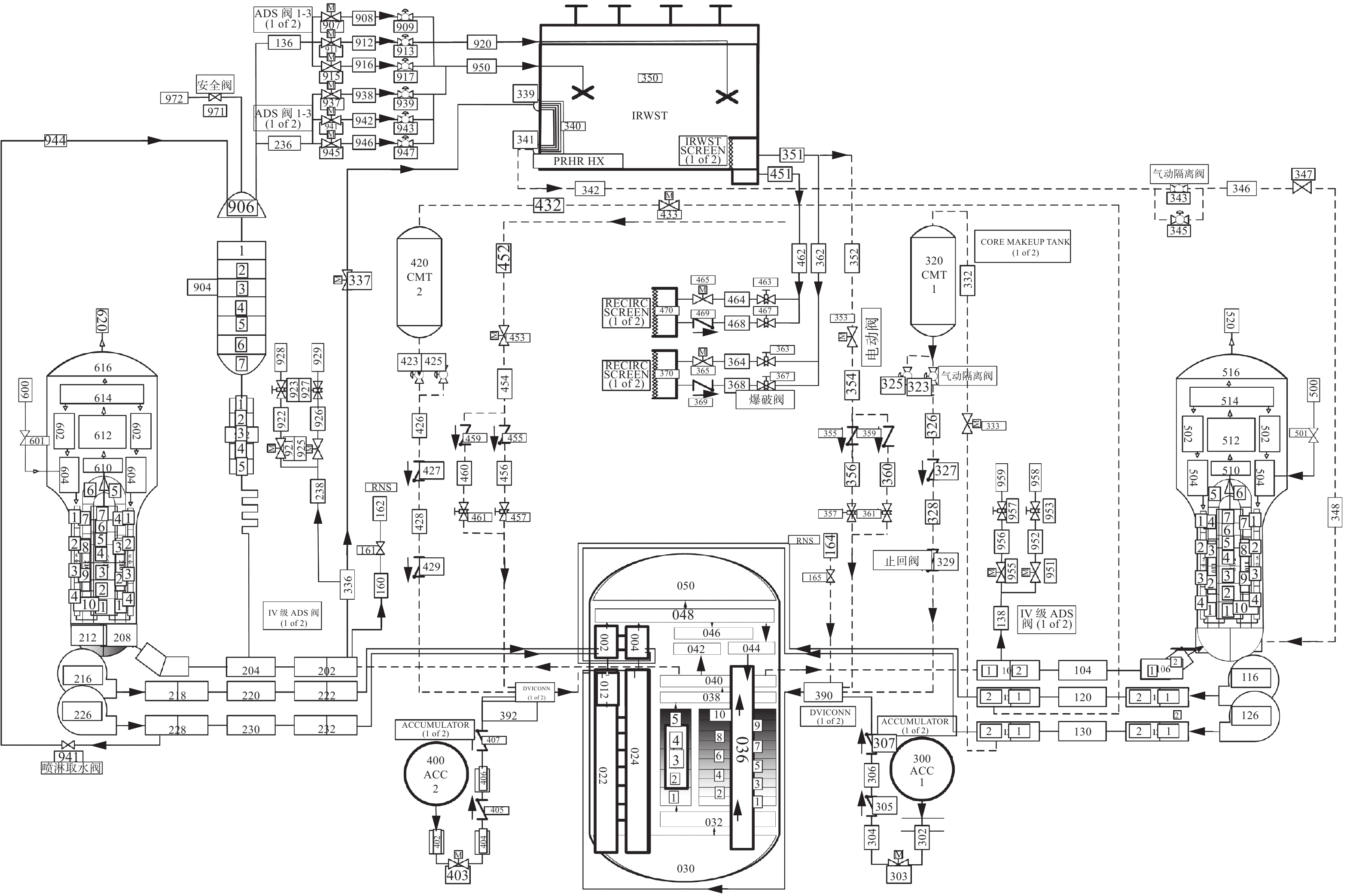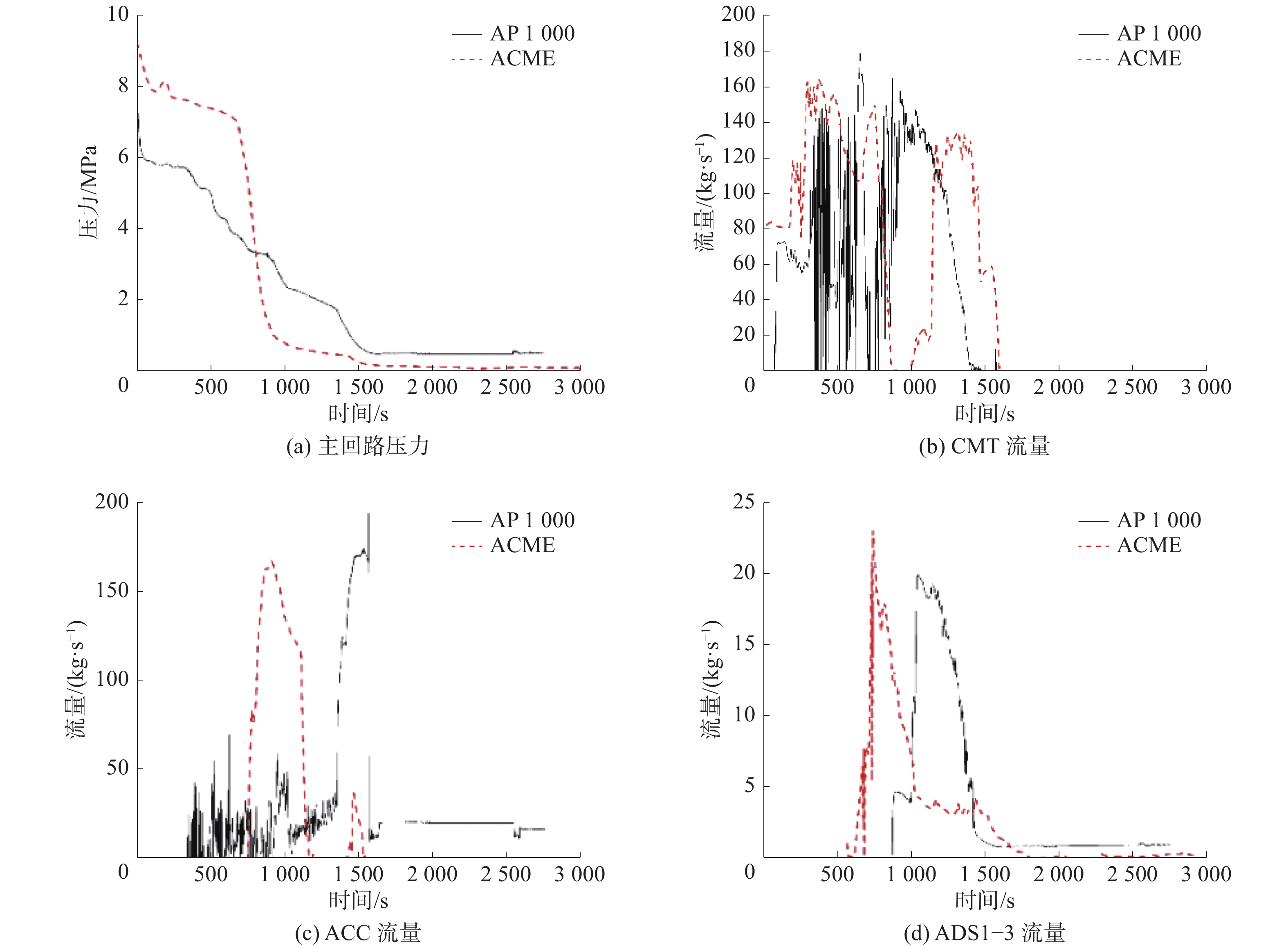﻿ SBLOCA整体试验台架的比例模化分析与初步评估
«上一篇文章快速检索 高级检索

 应用科技2019, Vol. 46Issue (5): 80-87  DOI: 10.11991/yykj.2018120020

### 引用本文LU Xia, KUANG Bo, KONG Haozheng, et al. Scaling analysis and preliminary evaluation of integral effect test facility for SBLOCA[J]. Applied Science and Technology, 2019, 46(5): 80-87. DOI: 10.11991/yykj.201812002.### 文章历史

SBLOCA整体试验台架的比例模化分析与初步评估

Scaling analysis and preliminary evaluation of integral effect test facility for SBLOCA
LU Xia , KUANG Bo, KONG Haozheng , LIU Pengfei
School of Mechanical Engineering, Shanghai Jiao Tong University, Shanghai 200240, China
Abstract: In order to verify the suitability of safety design of large passive advanced pressurized water reactor (PWR) and the reliability of related accident analysis program, the suitability of modeling the AP1000 under small break loss of coolant accident (SBLOCA) through the Advanced Core-cooling Mechanism Experiment (ACME) is evaluated in this paper. The process of SBLOCA with medium and high importance in the phenomena identification and ranking table (PIRT) is verified by means of hierarchical two-tiered scaling (H2TS). In addition, the system analysis program RELAP5 is used to scale the SBLOCA process of the AP1000. The scaling results are compared with ACME test results, to evaluate the suitability of using ACME to scale the SBLOCA of AP1000.
Keywords: large passive advanced pressurized water reactor    SBLOCA    PIRT    H2TS    integral effect test facility    ACME    scaling analysis    RELAP5 program calculation

1 大型非能动先进压水堆SBLOCA的重要现象过程表 1 小破口失水事故进程中重要现象
2 大型非能动先进压水堆整体验证试验台架的模化设计要求

2.1 破口喷放阶段

2.2 自然循环阶段 2.2.1 Top-Down模化分析

 $\frac{{\partial {\rho _{\rm{m}}}}}{{\partial t}} + \frac{\partial }{{\partial z}}\left( {{\rho _{\rm{m}}} + {u_{\rm{m}}}} \right) = 0$

 \begin{aligned} \dfrac{{\partial {\rho _{\rm{m}}}{u_{\rm{m}}}}}{{\partial t}} + & \dfrac{\partial }{{\partial z}}\left( {{\rho _{\rm{m}}}u_{\rm{m}}^2} \right) = - \dfrac{{\partial {P_{\rm{m}}}}}{{\partial z}} - {\rho _{\rm{m}}}g - \dfrac{\partial }{{\partial z}}\left[ {\dfrac{{\alpha {\rho _{\rm{g}}}\rho }}{{\left( {1 - \alpha } \right){\rho _{\rm{m}}}}}V_{{\rm{gj}}}^2} \right] - \\ & \left[ {\dfrac{{{f_{\rm{m}}}}}{{2d}} + K\delta \left( {z - {z_i}} \right)} \right]{\rho _{\rm{m}}}{u_{\rm{m}}}\left| {\rho {u_{\rm{m}}}} \right| \end{aligned}

 \begin{aligned} \frac{{\partial {\rho _{\rm{m}}}{H_{\rm{m}}}}}{{\partial t}} + & \frac{\partial }{{\partial z}}\left( {{\rho _{\rm{m}}}{u_{\rm{m}}}{H_{\rm{m}}}} \right) = - \frac{{4{h_{\rm{m}}}}}{d}\left( {{T_{\rm{s}}} - {T_{{\rm{sat}}}}} \right) - \\ & \frac{\partial }{{\partial z}}\left[ {\frac{{\alpha {\rho _{\rm{g}}}\rho }}{{{\rho _{\rm{m}}}}}{\rm{\Delta }}{H_{{\rm{fg}}}}{V_{{\rm{gj}}}}} \right] \\ \end{aligned}

 $\begin{gathered} {\pi _{\rm{R}}} = {\left[ {{x_{\rm{e}}} \cdot \left( {\frac{{\Delta \rho }}{{{\rho _g}}}} \right)} \right]_{\rm{R}}} \\ {u_{\rm{R}}}{\rm{ = }}\sqrt {{l_{\rm{R}}}} \\ {q_{\rm{R}}} = 1/\sqrt {{l_{\rm{R}}}} \\ \end{gathered}$

2.2.2 Bottom-Up模化分析

 $Fr = \frac{{{j_{\rm{g}}}}}{{\sqrt {gd} }}{\left( {\frac{{{\rho _{\rm{g}}}}}{{{\rho _{\rm{f}}} - {\rho _{\rm{g}}}}}} \right)^{1/2}}$

 ${\left( {{\pi _{Fr}}} \right)_{\rm{R}}} = {\left[ {\frac{{{q_{{\rm{core}}}}}}{{{d_{{\rm{CL}}}}^{5/2}}}} \right]_{\rm{R}}}$

 $\frac{{{X_{{\rm{branch}}}}}}{{{X_{{\rm{main}}}}}} = {\left( {\frac{{{G_{{\rm{branch}}}}}}{{{G_{{\rm{main}}}}}}} \right)^{ - 0.8}}$

 ${\left( {{\pi _x}} \right)_{\rm{R}}} = \left[ {{{\left( {\frac{{{d_{{\rm{CL}}}}}}{{{d_{{\rm{CMT, bl}}}}}}} \right)}^2}} \right]_{\rm{R}}^{ - 0.8}$

 $\left( {\frac{{{V_{{\rm{RCS, sv}}}}}}{{\left( {\gamma - 1} \right)}}} \right)\frac{{{\rm{d}}{P_{{\rm{RCS, sv}}}}}}{{{\rm{d}}t}} = {\dot m_{{\rm{core, s}}}}{h_{\rm{g}}} - {\dot m_{{\rm{ADS, s}}}}{h_{\rm{g}}}$

 ${\omega _{{\rm{core, s}} - {\rm{RCS, sv}}}} = {\left[ {\frac{{{{\dot m}_{{\rm{core, s}}}} \cdot {h_{\rm{g}}}}}{{{{\left( {V\Delta P} \right)}_{{\rm{RCS, s}}}}}}} \right]_{\rm{R}}}$
 ${\omega _{{\rm{ADS, s}} - {\rm{RCS, sv}}}} = {\left[ {\frac{{{{\dot m}_{{\rm{ADS, s}}}} \cdot {h_{\rm{g}}}}}{{{{\left( {V\Delta P} \right)}_{{\rm{RCS, s}}}}}}} \right]_{\rm{R}}}$

2.3.2 Bottom-Up模化分析

1）子阶段1: CMT注入主导阶段

 $\frac{{{\rm{d}}{M_{{\rm{vessel}}}}}}{{{\rm{d}}t}} = {\dot m_{{\rm{CMT}}}} - {\dot m_{{\rm{core}}}}$

 ${\rho _{\rm{f}}}g\left[ {{Z_{{\rm{CMT}}}} - {Z_{{\rm{DVI}}}}} \right] = \left[ {{{\left( {\sum \frac{R}{{{A^2}}}} \right)}_{{\rm{CMT - DVI}}}}} \right]\frac{{\dot m_{{\rm{CMT}}}^2}}{{2{\rho _{\rm{f}}}}}$

 ${q_{{\rm{core}}}} = {\dot m_{{\rm{core}}}}\left( {{h_{{\rm{out}}}} - {h_{{\rm{in}}}}} \right) = {\dot m_{{\rm{core}}}}\left( {{h_{{\rm{sub}}}} - {x_{{\rm{e}}}}{h_{{\rm{fg}}}}} \right)$

 ${\rho _{\rm{f}}}\frac{{{\rm{d}}{V_{{\rm{vl}}}}}}{{{\rm{d}}t}} = {\left[ {\frac{{2{\rho _{\rm{f}}}^2g\left[ {{Z_{{\rm{CMT}}}} - {Z_{{\rm{DVI}}}}} \right]}}{{{{\left( {\displaystyle\sum \dfrac{R}{{{A^2}}}} \right)}_{{\rm{CMT - DVI}}}}}}} \right]^{1/2}} - \dfrac{{{q_{{\rm{core}}}}}}{{(\Delta {h_{{\rm{sub}}}} + {x_{{\rm{e}}}}{h_{{\rm{f}}}})}}$

 $\begin{array}{l} \dfrac{{{\rho ^ + }{\rm{d}}V_{vl}^ + }}{{{\rm{d}}t}} = {\omega _{{\rm{CMT, vl}}}} \cdot \dot m_{{\rm{CMT}}}^ + - {\omega _{{\rm{core, vl}}}} \cdot \dot m_{{\rm{core}}}^ + \\ {\omega _{{\rm{CMT, vl}}}} = \dfrac{{\left[ {\dfrac{{2{\rho _{\rm{f}}}^2g\left[ {{Z_{{\rm{CMT}}}} - {Z_{{\rm{DVI}}}}} \right]}}{{{{\left( {\displaystyle\sum \dfrac{R}{{{A^2}}}} \right)}_{{\rm{CMT - DVI}}}}}}} \right]_{{\rm{ref}}}^{1/2}}}{{{{\left( {{\rho _{\rm{f}}}\Delta {V_{{\rm{vl}}}}} \right)}_{{\rm{ref}}}}}} \\ {\omega _{{\rm{core, vl}}}} = \dfrac{{{{\left[ {\dfrac{{{q_{{\rm{core}}}}}}{{\Delta {h_{{\rm{sub}}}} + {x_{{\rm{exit}}}}{h_{{\rm{fg}}}}}}} \right]}_{{\rm{ref}}}}}}{{{{\left( {{\rho _{\rm{f}}}\Delta {V_{{\rm{vl}}}}} \right)}_{{\rm{ref}}}}}} \end{array}$

2）子阶段2：IRWST注入主导阶段

 $\frac{{{\rm{d}}{M_{{\rm{vl}}}}}}{{{\rm{d}}t}} = {\dot m_{{\rm{IRWST}}}} - {\dot m_{{\rm{core}}}}$

 $\begin{array}{l} {\rho _{\rm{f}}}g\left[ {{Z_{{\rm{IRWST}}}} - {Z_{{\rm{DVI}}}}} \right] - \left[ {{P_{{\rm{RCS}}}} - {P_{{\rm{CONT}}}}} \right] = \\ \quad \quad \quad \quad \;\; \;\left[ {{{\left( {\displaystyle\sum \dfrac{R}{{{A^2}}}} \right)}_{{\rm{IRWST - DVI}}}}} \right]\dfrac{{m_{{\rm{IRWST}}}^2}}{{2{\rho _{\rm{f}}}}} \end{array}$

 ${q_{{\rm{core}}}} = {\dot m_{{\rm{core}}}}\left( {{h_{{\rm{out}}}} - {h_{{\rm{in}}}}} \right) = {\dot m_{{\rm{core}}}}\left( {{h_{{\rm{sub}}}} - {x_{{\rm{exit}}}}{h_{{\rm{fg}}}}} \right)$

 $\begin{array}{l} \dfrac{{{\rho ^ + }{\rm{d}}V_{{\rm{vl}}}^ + }}{{{\rm{d}}t}} = {\omega _{{\rm{IRWST-vl}}}} \cdot \dot m_{{\rm{IRWST}}}^ + - {\omega _{{\rm{core\_vl}}}} \cdot \dot m_{{\rm{core}}}^ + \\ {\omega _{{\rm{CMT - vl}}}} = \\ \quad \quad\quad \;\; \dfrac{{{{\left[ {\dfrac{{2{\rho _{\rm{f}}}\left[ {{\rho _{\rm{f}}}g\left( {{Z_{{\rm{IRWST}}}} - {Z_{{\rm{DVI}}}}} \right) - {\rho _{\rm{f}}}g\left( {{Z_{{\rm{PZR}}}} - {Z_{{\rm{HL}}}}} \right)} \right]}}{{{{\left( {\displaystyle\sum \dfrac{R}{{{A^2}}}} \right)}_{{\rm{IRWST - DVI}}}}}}} \right]}^{1/2}}}}{{{{\left( {{\rho _{\rm{f}}}\Delta {V_{{\rm{vl}}}}} \right)}_{{\rm{ref}}}}}}\\ {\omega _{{\rm{CMT - vl}}}} = \dfrac{{{{\left[ {\dfrac{{{q_{{\rm{core}}}}}}{{(\Delta {h_{{\rm{sub}}}} + {x_{{\rm{exit}}}}{h_{{\rm{fg)}}}}}}} \right]}_{{\rm{ref}}}}}}{{{{\left( {{\rho _{\rm{f}}}\Delta {V_{{\rm{vl}}}}} \right)}_{{\rm{ref}}}}}} \end{array}$
2.4.2 Bottom-Up模化分析

 ${\left( {{\pi _{Fr}}} \right)_{\rm{R}}} = {\left[ {\frac{{{q_{{\rm{core}}}}}}{{{d_{{\rm{HL}}}}^{5/2}}}} \right]_{\rm{R}}}$

 $\frac{{{u_{\rm{g}}}\sqrt {{\rho _{\rm{g}}}} }}{{({\rho _{\rm{f}}} - {\rho _{\rm{g}}}){L_{\rm{g}}}}} \geqslant 5.7{\left( {\frac{{{L_{\rm{g}}}}}{{{d_{{\rm{offtake}}}}}}} \right)^{3/2}}$ (1)

 ${\pi _{\rm{R}}} = {\left[ {\frac{{{u_{\rm{g}}}\sqrt {{\rho _{\rm{g}}}} }}{{\sqrt {g\left( {{\rho _{\rm{f}}} - {\rho _{\rm{g}}}} \right){L_{\rm{g}}}} }}} \right]_R}{\left( {\frac{{{d_{{\rm{offtake}}}}}}{{{L_{\rm{g}}}}}} \right)^{3/2}}$

 ${u_{\rm{g}}} = \frac{{{q_{{\rm{core}}}}}}{{{\rho _{\rm{g}}}{h_{{\rm{fg}}}}{A_{{\rm{offtake}}}}}} = \frac{{{q_{{\rm{core}}}}}}{{{\rho _{\rm{g}}}{h_{{\rm{fg}}}}{A_{{\rm{ADS}} - 4}}}}$

 ${\pi _{\rm{R}}} = {\left[ {\frac{{{q_{{\rm{core}}}}}}{{{d_{{\rm{ADS}} - 4{\rm{inlet}}}}^{1/2} \cdot d_{{\rm{HL}}}^2}}} \right]_{\rm{R}}}$

 $K_{\rm{i}}^* = \frac{{\rho _{\rm{i}}^{1/2}{j_{\rm{i}}}}}{{{{(({\rho _{\rm{f}}} - {\rho _{\rm{g}}})g{\rm{\sigma }})}^{1/4}}}}$

 ${\pi _{\rm{R}}} = {\left[ {\frac{{{j_{\rm{g}}}{{(\rho _{\rm{f}}^{1/4} + \rho _{\rm{g}}^{1/4})}^2}}}{{\Delta \rho {\rm{\sigma }}}}} \right]_{\rm{R}}}$

 ${\pi _{\rm{R}}} = {\left[ {{{\left( {{j_{\rm{g}}}} \right)}_{{\rm{SL}}}}} \right]_{\rm{R}}} = {\left[ {{{\left( {{j_{\rm{f}}}} \right)}_{{\rm{SL}}}}} \right]_{\rm{R}}}$

2.5 IRWST注入阶段 2.5.1 Top-Down模化分析

 $\dot m = {\rm{constant}}$

 $\begin{gathered} g{\rho _{\rm{L}}}\left[ {{Z_{{\rm{IRWST}}}} - {Z_{{\rm{core, i}}}}} \right] - g{{\bar \rho }_{\rm{L}}}\left[ {{Z_{{\rm{core}}, 2\phi }} - {Z_{{\rm{core, i}}}}} \right] - \\ g{{\bar \rho }_{\rm{m}}}\left[ {{Z_{{\rm{IRWST}}}} - {Z_{{\rm{core}}, 2\phi }}} \right] = \\ \left( {\frac{{{{\dot m}^2}}}{{2{\rho _{\rm{f}}}}}} \right)\left[ {{{\left( {\sum \frac{R}{{{A^2}}}} \right)}_{{\rm{DVI}}}} + \phi _{{f_0}}^2{{\left( {\sum \frac{R}{{{A^2}}}} \right)}_{{\rm{ADS}}}}} \right] \\ \end{gathered}$

 \begin{aligned} {q_{{\rm{core}}}} = & \dot m\left( {{h_{{\rm{core, o}}}} - {h_{{\rm{core, i}}}}} \right) = \dot m\left[ {\left( {{h_{\rm{f}}} - {x_{{\rm{e}}}}{h_{{\rm{fg}}}}} \right) - {h_{\rm{L}}}} \right] = \\ & \dot m\left[ {\Delta {h_{{\rm{sub}}}} + {x_{{\rm{e}}}}{h_{{\rm{fg}}}}} \right] \\ \end{aligned}

 ${\pi _{\rm{R}}} = {\left[ {{x_e}} \right]_{\rm{R}}}$
2.5.2 Bottom-Up模化分析

 $\alpha = 0.925{\left( {\frac{{{\rho _{\rm{g}}}}}{{{\rho _{\rm{f}}}}}} \right)^{0.24}}{\left( {\frac{{{j_{\rm{g}}}}}{{{V_{{\rm{bcr}}}}}}} \right)^a}{\left( {\frac{{{j_{\rm{g}}}}}{{{j_{\rm{g}}} + {j_{\rm{f}}}}}} \right)^{0.6}}$ (2)

 ${j_{\rm{g}}}\left( Z \right) = \frac{{{q_{{\rm{core}}}} \cdot Z}}{{{\rho _{\rm{g}}}{h_{{\rm{fg}}}}{L_{{\rm{core}}}}{A_{{\rm{core}}}}}} = \frac{{{q_{{\rm{core}}}}}}{{{V_{{\rm{core}}}}}} \cdot \frac{Z}{{{\rho _{\rm{g}}}{h_{{\rm{fg}}}}}}$ (3)

 ${\pi _{\rm R}} = {\left( \alpha \right)_{\rm R}} = \left( {\frac{{{q_{{\rm{core}}}} \cdot Z}}{{{V_{{\rm{core}}}}}}} \right)_{\rm R}^{0.57}$
2.6 地坑注入阶段

3 ACME台架模拟AP1000核电厂的模化评估

ACME台架是以大型非能动先进压水堆为原型，通过比例分析设计的整体试验台架。其与一般的非能动压水堆相比，最大的区别是有2个安全壳内置换料水箱，其中IRWST−1水箱直径较大，高度为电厂原型的1/3，作为热阱；IRWST−2水箱直径较小，高度与电厂原型相同，作为重力注射的水源

ACME台架与AP1000电厂根据模化设计要求所得到的模化比总结于表2中。对于与出口干度、空泡份额等相关的模化比可以通过对功率和阻力的预计算及实验过程中的调试来保证；对于与几何尺寸、堆芯功率等相关的模化比，可以根据表3中所示ACME与AP1000电厂的重要参数比计算出。表 2 ACME/AP1000的SBLOCA事故进程中各个阶段的模化比表 3 ACME与AP1000重要参数比

4 ACME台架实验值与AP1000电厂RELAP5计算值结果比较

2-inch小破口失水事故是非能动压水堆小破口失水事故的典型工况，因此，本文使用RELAP5系统分析程序模拟AP1000反应堆冷管段2-inch小破口失水事故进程，并将计算结果与ACME台架的实验数据对比，从具体事故进程角度验证ACME台架是否能很好地模拟AP1000。图1所示为应用Relap5程序对AP1000建模时的节点划分图。为了更加直观地对比ACME台架实验结果和AP1000的计算结果，按照表2中的时间等参数的比例关系，把ACME台架的实验结果折算后与AP1000的计算结果放在同一图上图2显示了冷段小破口工况下主回路压力和3个非能动安全设备注入流量随时间的变化情况。需注意，ACME台架稳态条件模拟的起点所对应于真实AP1000发电厂的瞬态点是当AP1000的主系统压力降至9.2 MPa时。Download: 图 1 AP1000 RELAP5节点Download: 图 2 冷段小破口工况下主回路压力和3个非能动安全设备注入流量随时间的变化

5 结论

1）本文基于H2TS系统分析方法，对大型非能动先进压水堆进行自上而下和自下而上的比例分析，得到了整体试验台架模拟原型压水堆需要满足的模化比。

2）结合ACME台架与AP1000电厂设计的重要参数比，计算ACME模拟AP1000的模化比，验证了ACME从比例分析角度模拟AP1000的SBLOCA进程是适宜的。

3）通过对ACME台架的实验结果与AP1000小破口工况的计算结果进行对比分析，表明台架的非能动系统设计合理，能较好地模拟并验证非能动压水堆相关事故中的系统响应。

  Westinghouse Electric Company LLC. AP1000 PIRT and scaling assessment[R]. 15230−0355. Pittsburgh, PA, 2001. (0)  ZUBER N. Appendix D: hierarchical, two-tiered scaling analysis, an integrated structure and scaling methodology for severe accident technical issue resolution. NUREG/CR-5809[R]. Washington: U.S Nuclear Regulatory Commission, 1991. (0)  ISHII M, KATAOKA I. Scaling laws for thermal-hydraulic system under single phase and two-phase natural circulation[J]. Nuclear engineering and design, 1984, 81(3): 411-425. DOI:10.1016/0029-5493(84)90287-5 (0)  TAITEL Y, DUKLER A E. A model for predicting flow regime transitions in horizontal and near horizontal gas-liquid flow[J]. AIChE journal, 1976, 22(1): 47-55. DOI:10.1002/(ISSN)1547-5905 (0)  SEEGER W, REIMANN J, MÜLLER U. Two-phase flow in a T-Junction with a horizontal inlet. Part I: phase separation[J]. International journal of multiphase flow, 1986, 12(4): 575-585. DOI:10.1016/0301-9322(86)90061-3 (0)  ZUBER N. Problems in modeling of small break LOCA[C]// Heat Transfer in Nuclear Reactor Safety. New York: Hemisphere Publishing, 1982. (0)  ZHU Sheng. Test analysis of CAP14005cm break accident based on the acme test facility[C]//Proceedings of the 201624th International Conference on Nuclear Engineering. USA, North Carolina, Charlotte, 2016. (0)  李玉全, 常华健, 叶子申, 等. ACME整体试验台架堆芯模拟体设计[J]. 动力工程学报, 2016, 36(3): 236-241. DOI:10.3969/j.issn.1674-7607.2016.03.012 (0)  汪映荣. CAP1400核电技术特点和工程进展[J]. 中国核电, 2018, 11(1): 35-40. (0)  邓程程, 常华健, 秦本科, 等. 非能动试验台架中CMT的比例分析及失真评价[J]. 原子能科学技术, 2013, 47(11): 2026-2032. DOI:10.7538/yzk.2013.47.11.2026 (0)  SCDAP/RELAP5 Development Team. SCDAP/RELAP5/MOD 3.2 code manual[R]. Washington: Idaho National Engineering Laboratory, 1998. (0)  朱升. ACME台架程序建模及试验初始条件确定方法研究[J]. 原子能科学技术, 2016, 50(7): 1179-1185. (0)